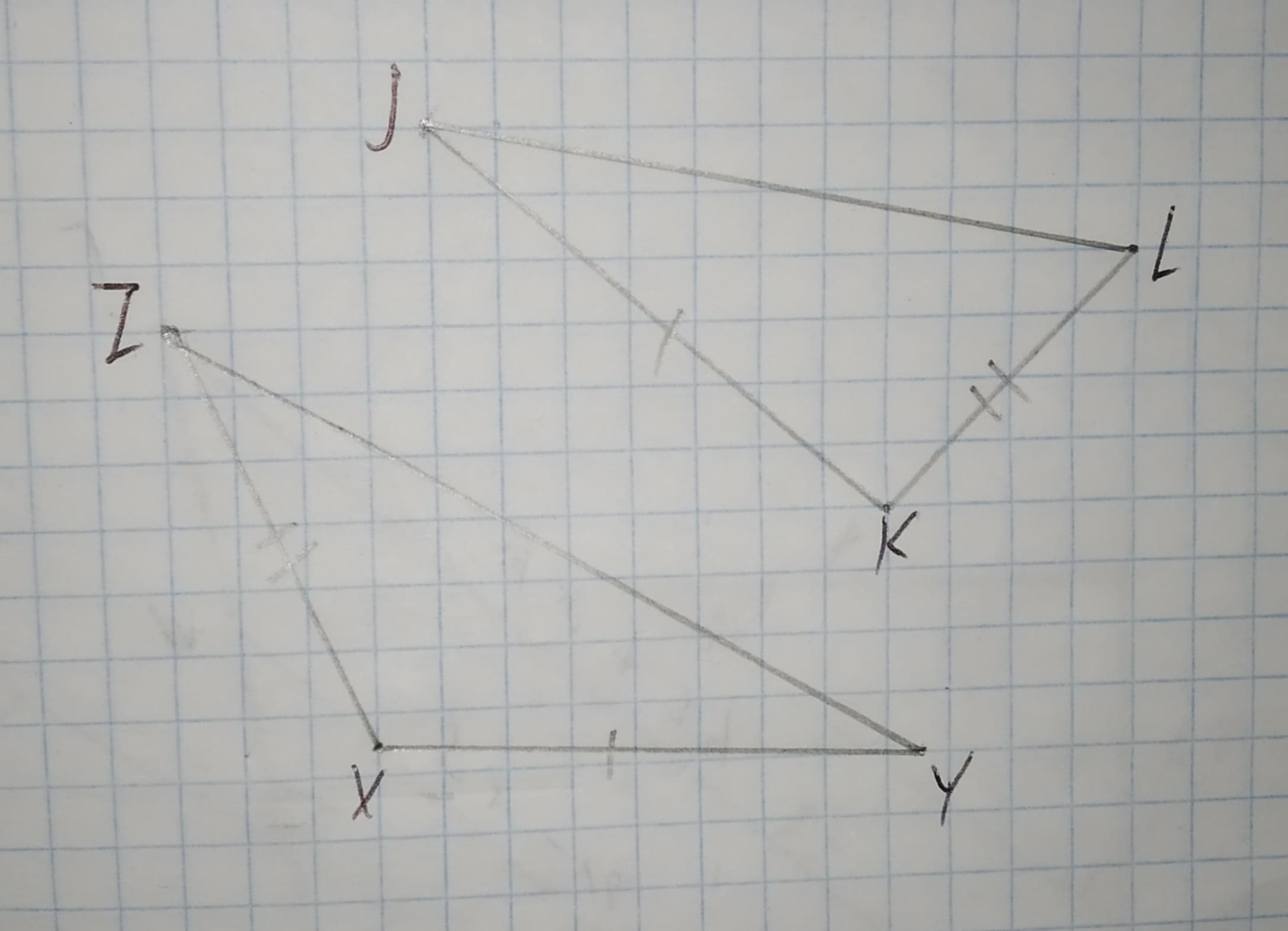# State the third congruence required to prove the congruence of triangles using the indicated postulate.12210203582.jpga)bar(ZY) ~= bar(JL)b)/_X ~= /_Kc)bar(KL) ~= bar(XZ)d)/_Y ~= /_LJason Farmer 2021-02-21 Answered

State the third congruence required to prove the congruence of triangles using the indicated postulate.a)$\stackrel{―}{ZY}\stackrel{\sim }{=}\stackrel{―}{JL}$
b)$\mathrm{\angle }X\stackrel{\sim }{=}\mathrm{\angle }K$
c)$\stackrel{―}{KL}\stackrel{\sim }{=}\stackrel{―}{XZ}$
d)$\mathrm{\angle }Y\stackrel{\sim }{=}\mathrm{\angle }L$

You can still ask an expert for help

## Want to know more about Congruence?

• Questions are typically answered in as fast as 30 minutes

Solve your problem for the price of one coffee

• Math expert for every subject
• Pay only if we can solve itestenutC

Step 1
Given figure,we have to state the congruence required to prove the congruence of triangles using the indicated postulate.
Step 2
It is given that both triangles are congruent by criteria of SAS
$In\mathrm{△}ZXY\phantom{\rule{1em}{0ex}}\text{and}\phantom{\rule{1em}{0ex}}\mathrm{△}LKJ$
$\stackrel{―}{ZX}\stackrel{\sim }{=}\stackrel{―}{KL}$
$\stackrel{―}{XY}\stackrel{\sim }{=}\stackrel{―}{JK}$
$\mathrm{\angle }X\stackrel{\sim }{=}\mathrm{\angle }K$
by criteria of SAS
$\mathrm{△}ZXY\stackrel{\sim }{=}\mathrm{△}LKJ$
so, the third congruence is $\mathrm{\angle }X\stackrel{\sim }{=}\mathrm{\angle }K$ to prove the congruence of triangles
so, option B is correct.